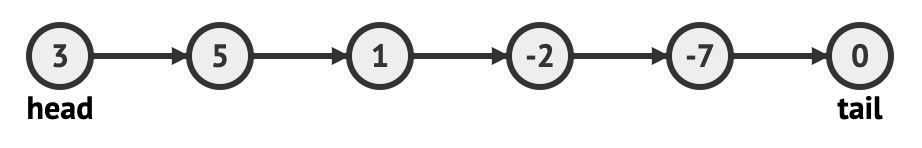Login or Create an Account to view the mark scheme, comment, and add to a test
A collection called NUMS is represented as follows:Consider the following pseudocode:
COUNTER = 0 SUM = 0 NUMS.resetNext() loop while NUMS.hasNext() N = NUMS.getNext() if N > 0 then COUNTER = COUNTER + 1 SUM = SUM + N end if end loop RESULT = SUM / COUNTER output RESULT
Trace the pseudocode above by tracking variables COUNTER, SUM, N and RESULT.

HomeThe Right Test - Fast